Home > INT3 > Chapter 11 > Lesson 11.2.2 > Problem11-68

11-68.
1. For each of the following equations, list every point where its three-dimensional graph intersects one of the coordinate axes. That is, what are the x‑, y‑, and z‑intercepts? Express your answers in (x, y, z) form. 11-68 HW eTool (CPM). Homework Help ✎

1. 6y + 15z = 60

2. 3x + 4y + 2z = 24

3. (x + 3)2 + z2 = 25

4. z = 6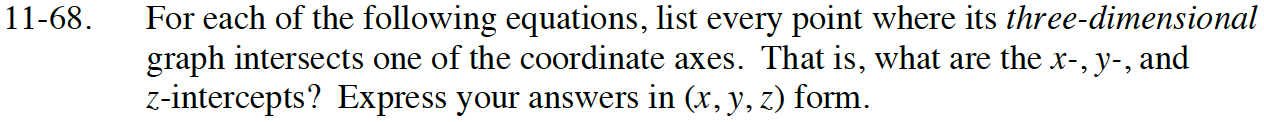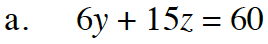To find the intercept of a given axis, set all other variables to zero.
For example, the y-intercept will be (0, ?, 0).
To find the y-intercept, let x = 0 and z = 0

Repeat the process to find the z-intercept. (0, 0, ?).

(0, 10, 0) and (0, 0, 4)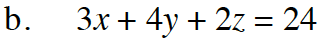See part (a):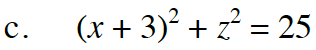See part (a).
Note: You will have two x-intercepts and two z-intercepts. Why?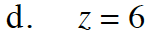See part (a):

Use the eTool below to test your solutions to parts (a), (b) and (c) above.
Click on the link at right for the full eTool version: INT3 11-68 HW eTool